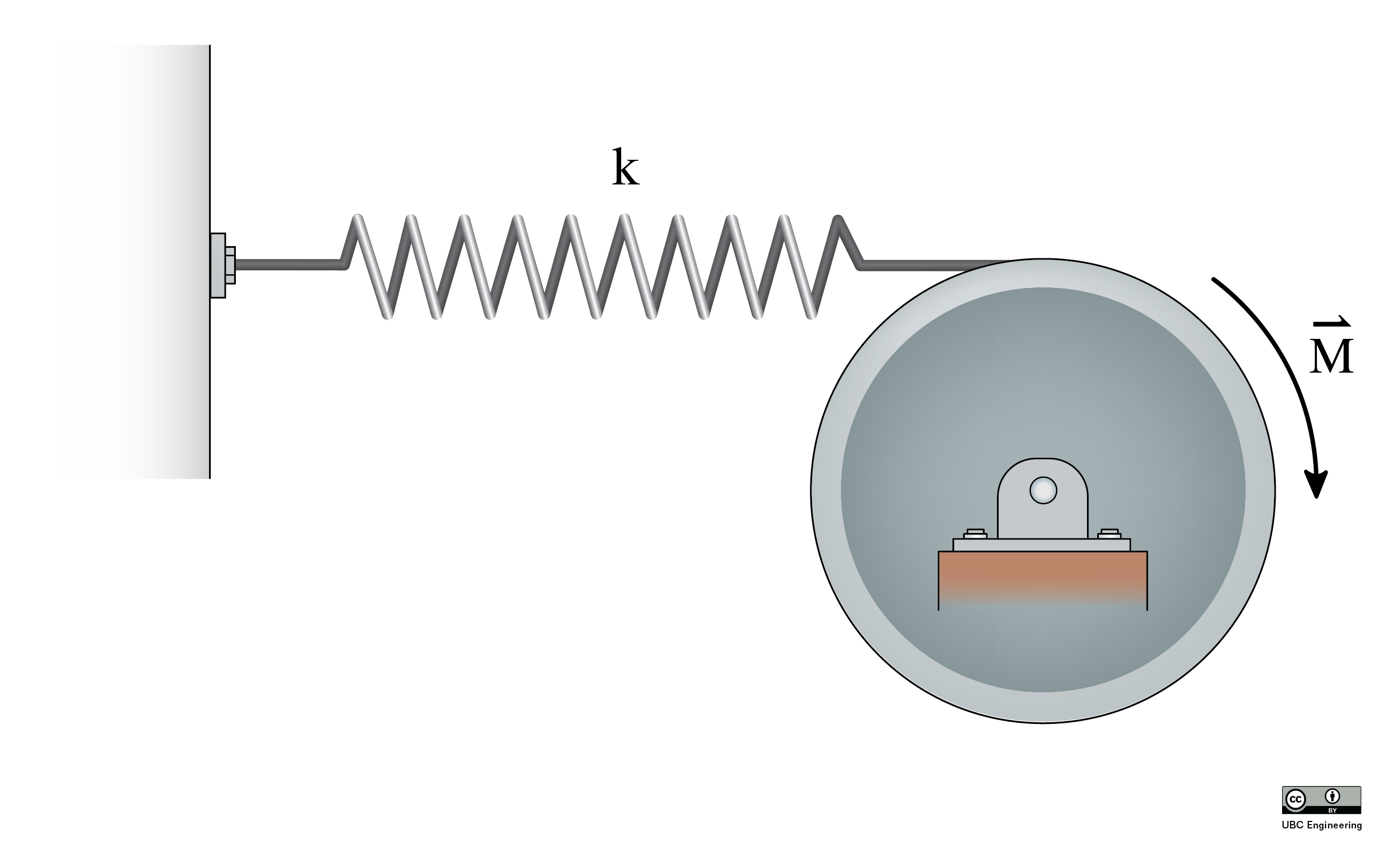﻿ Mechanics Map - Undamped Free Vibrations

# Undamped Free Vibrations

Vibrations occur in systems that attempt to return to their resting or equilibrium state when perturbed (or pushed away from their equilibrium state). The simplest vibrations to analyze are undamped, free, one degree of freedom vibrations.

"Undamped" means that there are no energy losses with movement (whether intentional, by adding dampers, or unintentional, through drag or friction). An undamped system will vibrate forever without any additional applied forces. A simple pendulum has very low damping, and will swing for a long time before stopping. ("Damped" means that there are resistive forces and energy losses with movement that cause the system to stop moving eventually.)

"Free" means that, after the initial perturbation, the only forces acting on the system are internal to the system (springs, dampers) and/or gravity. A tuning fork continues to vibrate after one initial perturbation (being struck). ("Forced" means there is an external, typically periodic, force acting on the system. A jackhammer vibrates due to having a supply of compressed air continually forcing the bit up and down, and it stops vibrating very quickly without that external periodic forcing).

"One degree of freedom" means that we will only consider systems with one mass vibrating along one direction (e.g. use variable x) or about one axis (e.g. use variable θ). Systems having more than one mass or vibrating along or about two or more axes have more than one degree of freedom.

We can derive the equation of the system by setting up a free-body diagram. Consider a mass sitting on a frictionless surface, attached to a wall via a spring.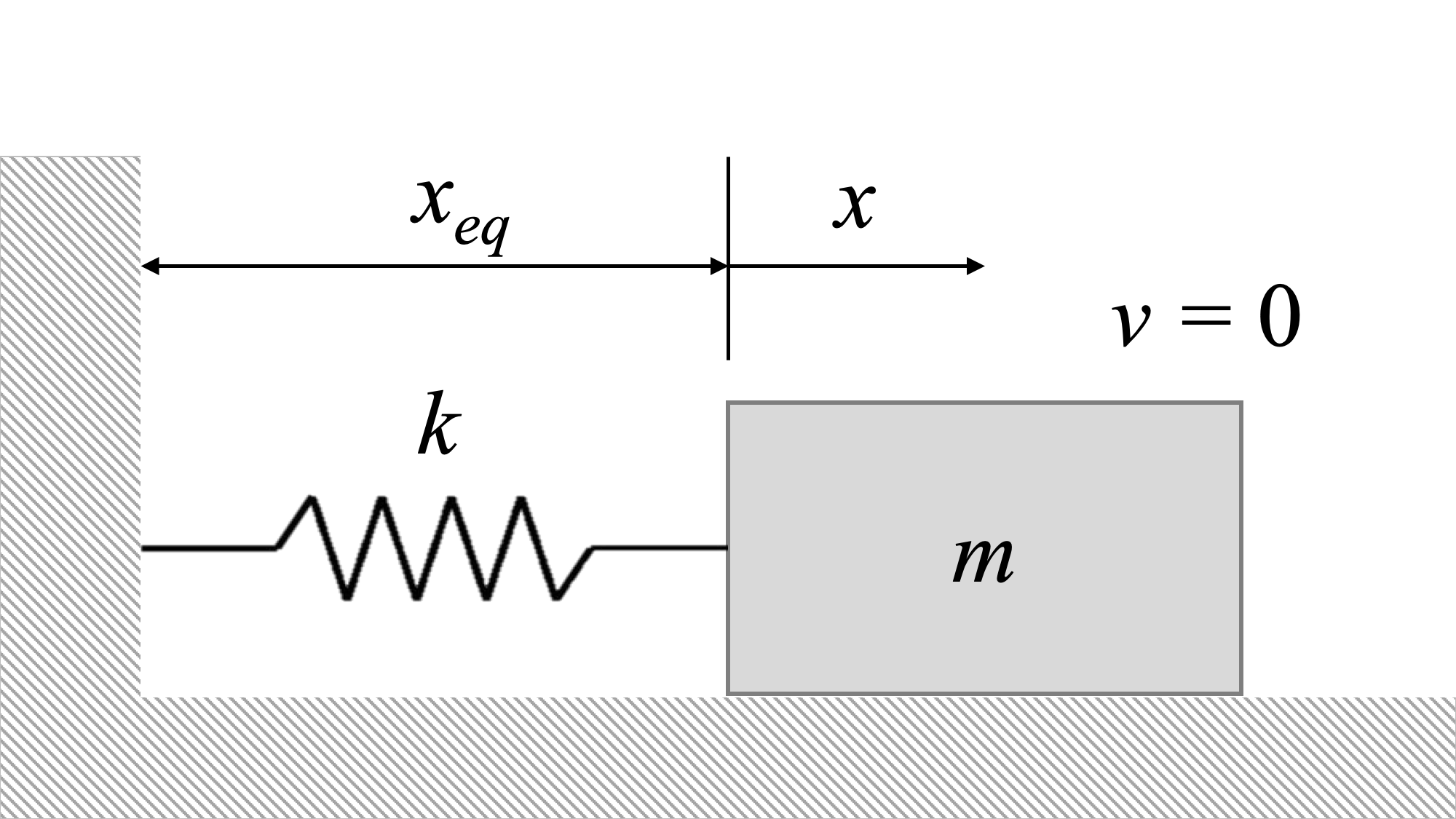This is a system consisting of a mass attached to the wall via a spring, sitting on a frictionless surface. The system is currently in equilibrium, and the spring is not stretched or compressed.

The system above is in equilibrium. It is at rest, and will stay at rest unless some other force acts on it.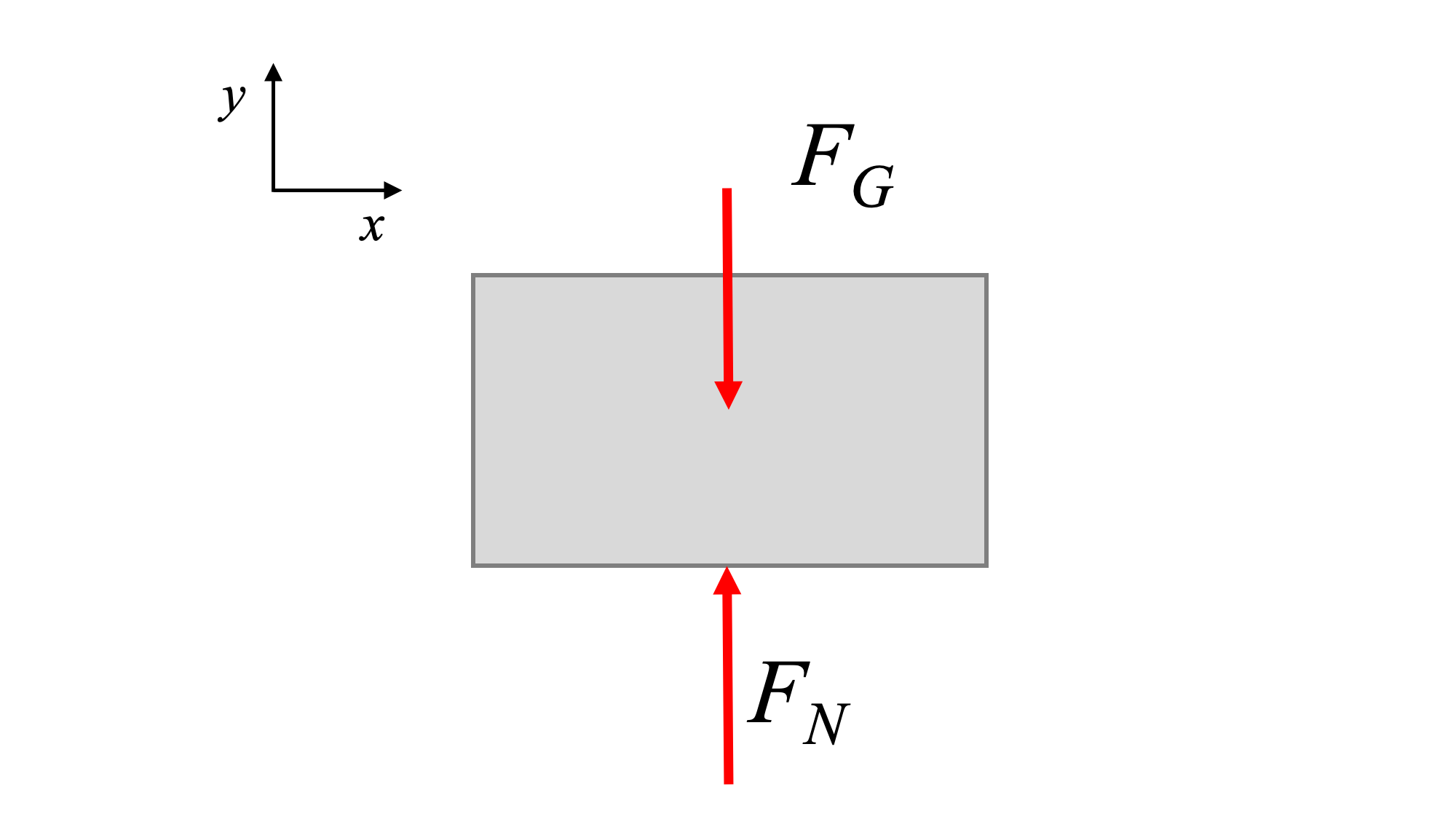Free-body diagram of the system in equilibrium position. Since the spring is at its unstretched length, it does not produce a force.

To start the system vibrating, we need to perturb it. Perturbation is moving the system away from equilibrium by a small amount.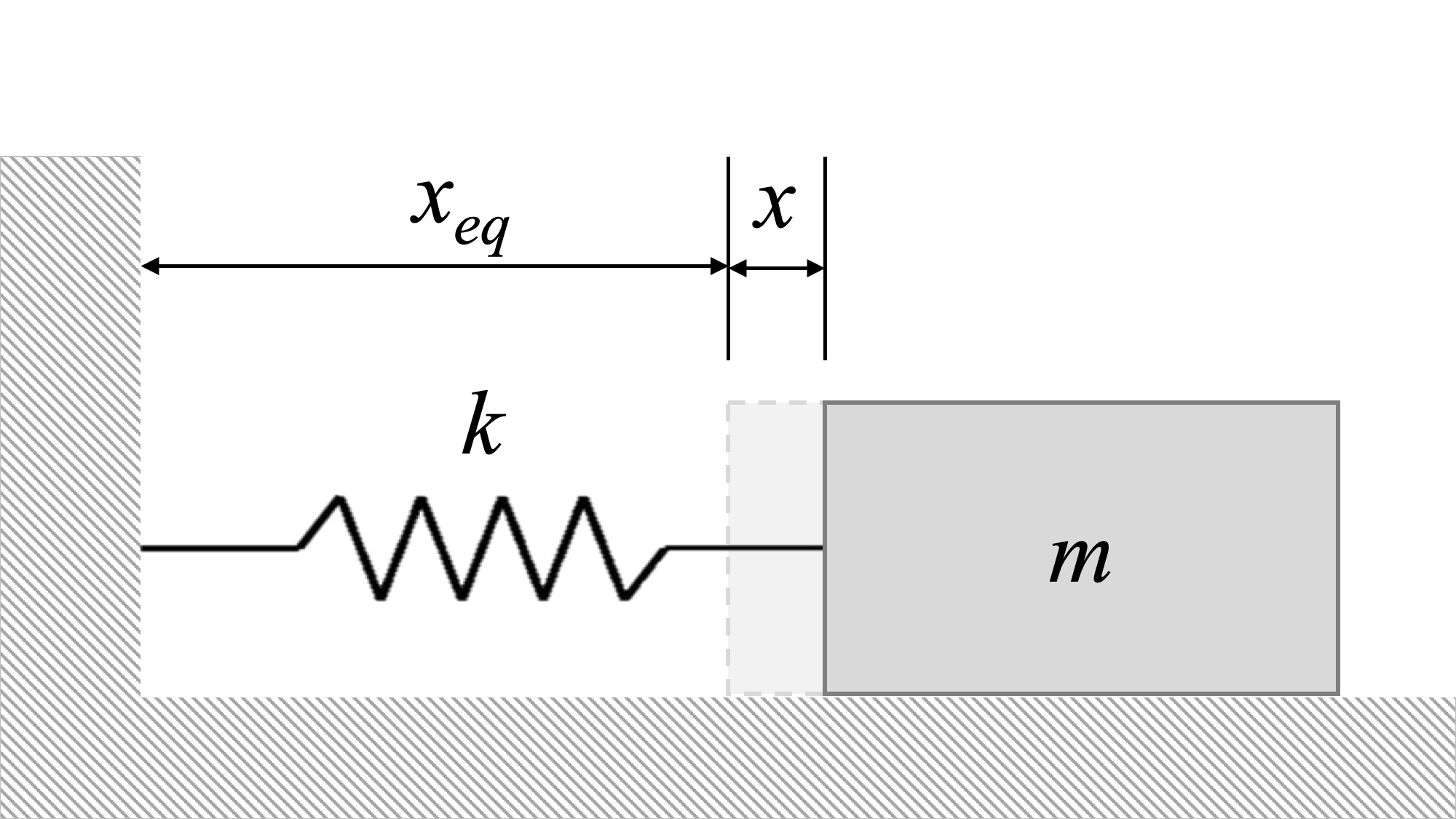Mass-spring system perturbed by an amount x from equilibrium. This stretches the spring, causing a spring force which tends to pull the mass back toward equilibrium.

When we perturb this system, we stretch the spring. This generates a spring force, and the spring force is always in a direction that tends to pull the system back toward equilibrium.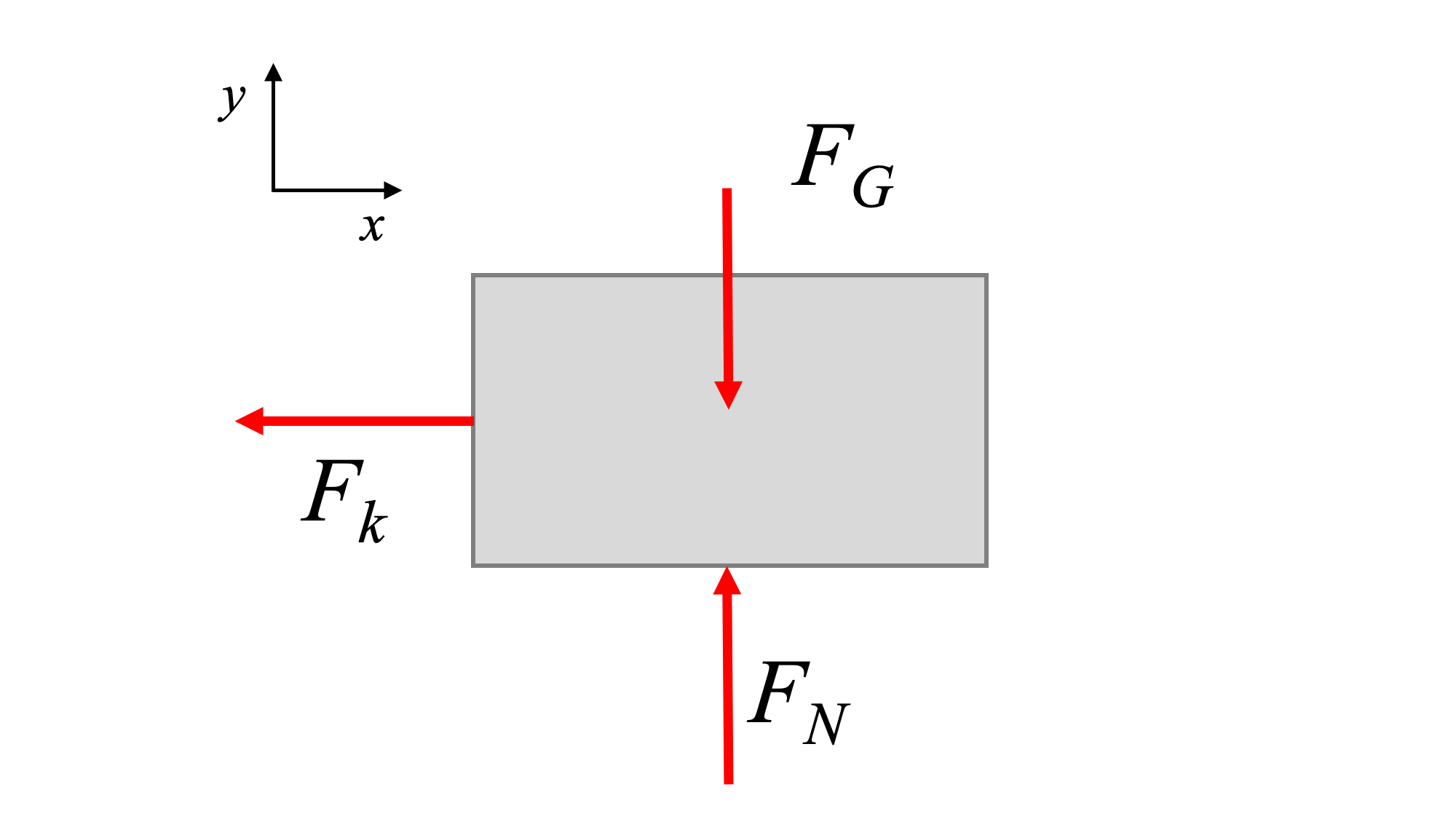Mass-spring system perturbed by an amount x from equilibrium. This stretches the spring, causing a spring force which tends to pull the mass back toward equilibrium.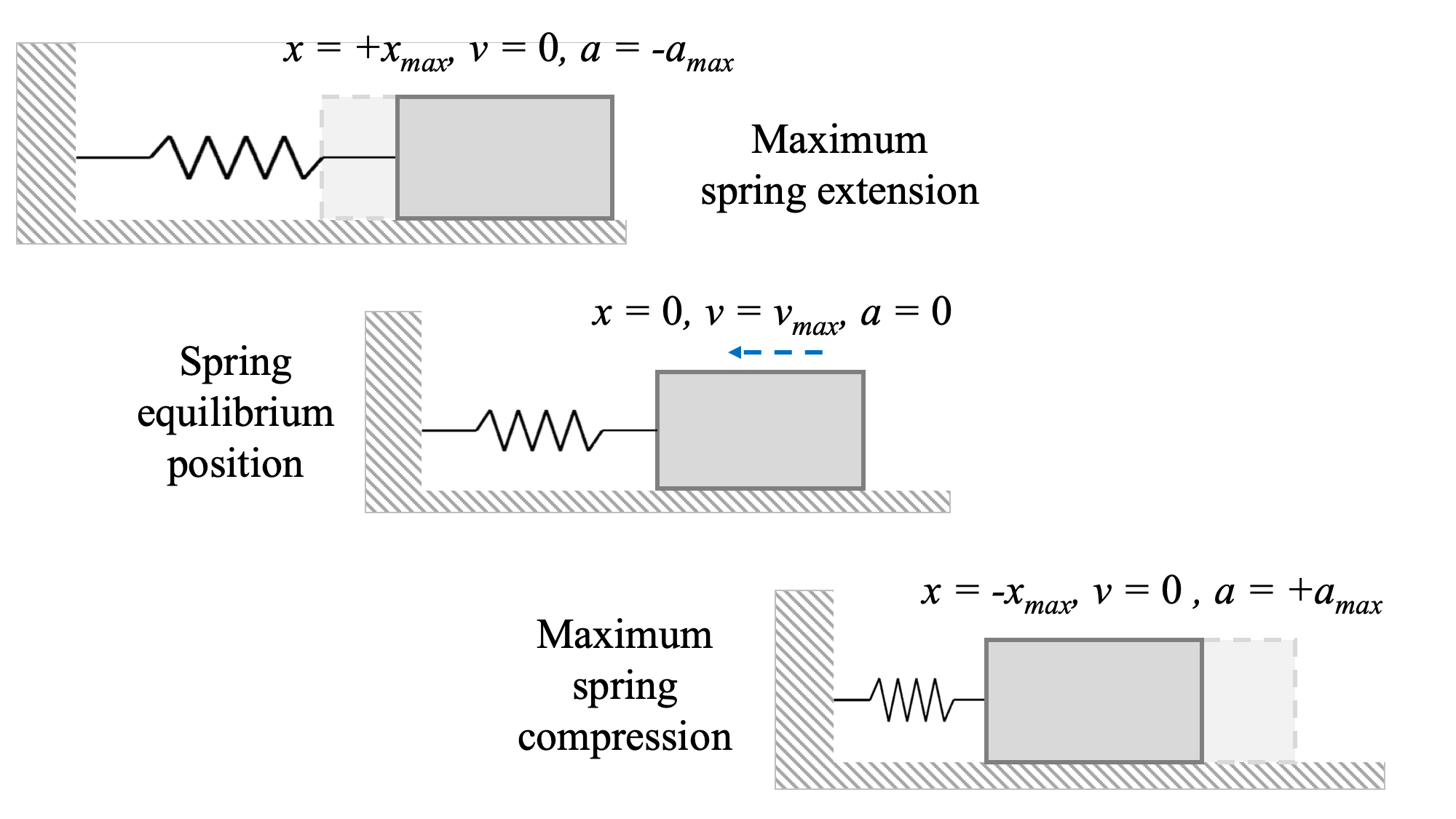Mass-spring system vibration. After an initial perturbation, the system oscillates between two extreme positions (-x_max and x_max). The extreme positions are turn-around points where the velocity is zero, but the spring force is maximum (maximum stretch/compression) and therefore the acceleration is maximum. As the system passes through the equilibrium position, the spring is no longer stretched (zero force, zero acceleration), but the velocity is maximum (this can be determined using conservation of energy) and the inertia of the system carries the mass past the equilibrium position. In the absence of damping or the application of another force, the system will oscillate forever.

We can generate the equation of motion of the system, and determine the specifics of how it will vibrate, by analyzing this perturbed state. Recall that the spring force or moment is:

 $\vec{F{_k}} =k \vec{x}$ $\vec {M{_k}}=k \vec{θ}$

Note that the spring constants in the above equations have different units, depending on whether the spring is linear (N/m) or torsional (N-m/rad), and that θ must be given in radians. The magnitude of the spring force depends on x, the distance from the spring unstretched length (not necessarily the equilibrium position of the system), and the same is true for moment of a torsional spring. The spring force or moment is in the direction/orientation opposite that of the displacement. That is, if you pull the mass to the right, the spring force points to the left.

The process for finding the equation of motion of the system is as follows:

1. Sketch the system with a small positive perturbation (x or θ).
2. Draw the free-body diagram of the perturbed system. Ensure that the spring force has a direction opposing the perturbation.
3. Find the one equation of motion for the system in the perturbed coordinate using Newton's Second Law. Keep the same positive direction for position, and assign positive acceleration in the same direction.
4. Move all terms of the equation to one side, and check that all terms are positive. If all terms are not positive, there is an error in the direction of displacement, acceleration, and/or spring force.

For the example system above, with mass m and spring constant k, we derive the following:

 $\sum F_x = m a_x = m {\ddot{x}}$ $-F_k = m {\ddot{x}}$ $-k x = m {\ddot{x}}$ $m {\ddot{x}} + k x = 0$

This gives us a differential equation that describes the motion of the system. We can rewrite it in normal form:

 $m {\ddot{x}} + k {x} = 0$ $\Rightarrow \, {\ddot{x}} + \frac{k}{m} {x} = 0$ $\Rightarrow \, {\ddot{x}} + \omega_n^2 {x} = 0$

The term ωn is called the angular natural frequency of the system, and has units of rads/s.

 $\omega_n^2 = \frac{k}{m}$ $\omega_n = \sqrt{\frac{k}{m}}$

Assuming that the initial perturbation of the system can be described by the position and velocity of the mass at t=0,

 $x(0) = x_0$ $v(0) = \dot{x}(0) = v_0$

The solution to the differential equation, that provides the position of the system at time t, x(t) is:

 $x(t) = C \sin (\omega_n t + \phi)$ $\text{where:} \,\, \omega_n = \sqrt{\frac{k}{m}}, \, C = \sqrt{(\frac{v_0}{\omega_n})^2 + x_0^2}, \, \phi = \tan^{-1} (\frac{x_0 \omega_n}{v_0})$

The amplitude, C, describes the maximum displacement during the oscillations (i.e. xmax) and the phase, ϕ, describes how the sine function is shifted in time.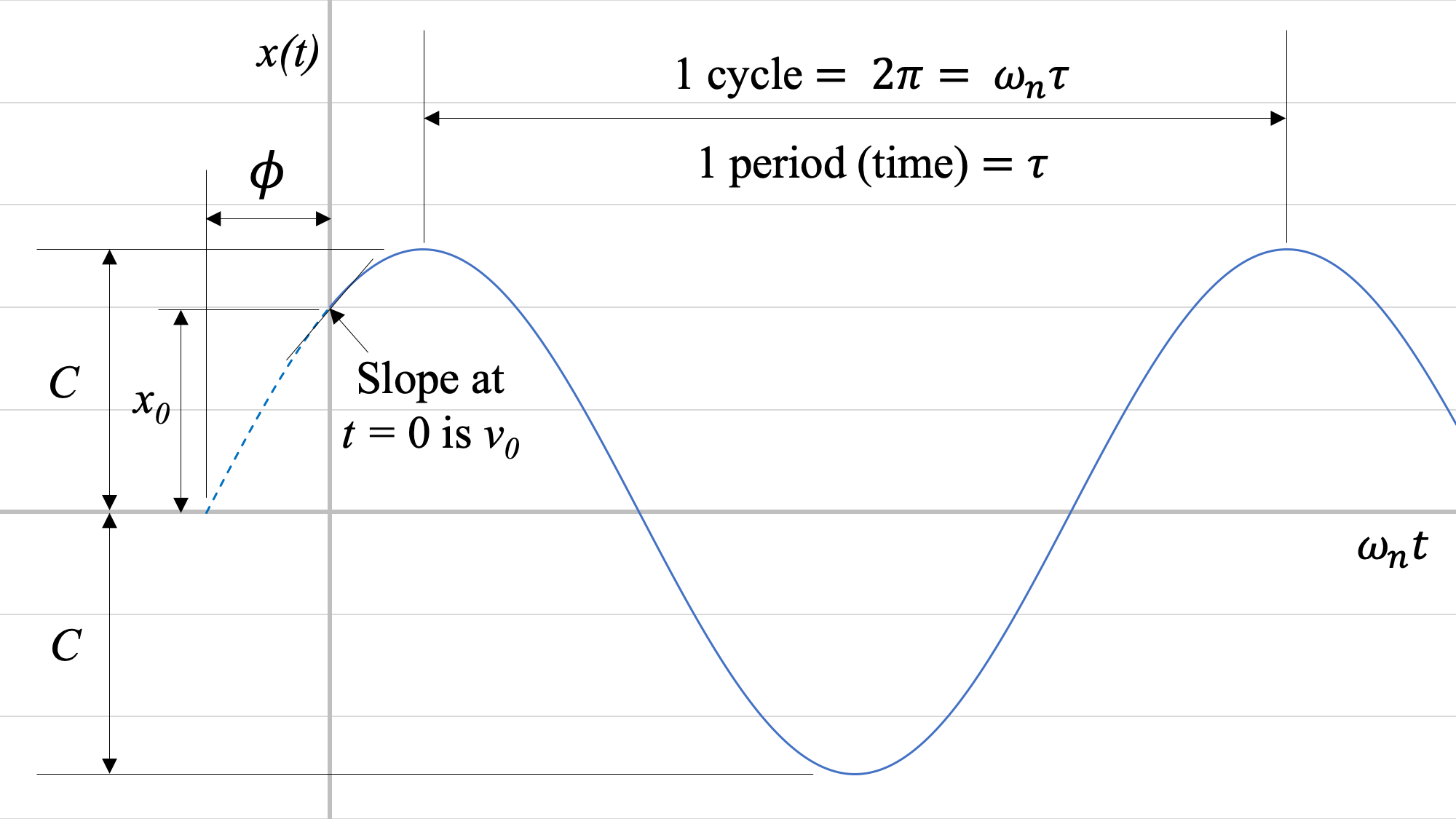Displacement response of the mass spring system (solution to the differential equation).

For a system where there is torsional vibration (that is, the oscillation involves a rotation), the equations are similarly:

 $I {\ddot{\theta}} + k{\theta} = 0$ $\Rightarrow \, {\ddot{\theta}} + \frac{k}{I}{\theta} = 0$ $\Rightarrow \, {\ddot{\theta}} + \omega_n^2 {\theta} = 0$ $\text{where:} \,\, \omega_n = \sqrt{\frac{k}{I}}$

Assuming that the initial perturbation of the system can be described by the position and velocity of the mass at t=0,

 $\theta (0) = \theta_0$ $\omega (0) = \dot{\theta} (0) = \omega_0$

The solution to the differential equation, that provides the position of the system at time t, θ(t) is:

 $\theta (t) = C \sin (\omega_n t + \phi)$ $\text{where:} \,\, \omega_n = \sqrt{\frac{k}{I}}, \, C = \sqrt{(\frac{\omega_0}{\omega_n})^2 + \theta_0^2}, \, \phi = \tan^{-1} (\frac{\theta_0 \omega_n}{\omega_0})$

## Worked Problems:

### Question 1:

Find an expression for the angular natural frequency of the following system, and find the maximum amplitude of vibration of the system with mass m = 10 kg and spring constant k = 200 N/m when given an inital displacement of x0 = 0.1 m and an initial velocity of v0 = 0.3 m/s.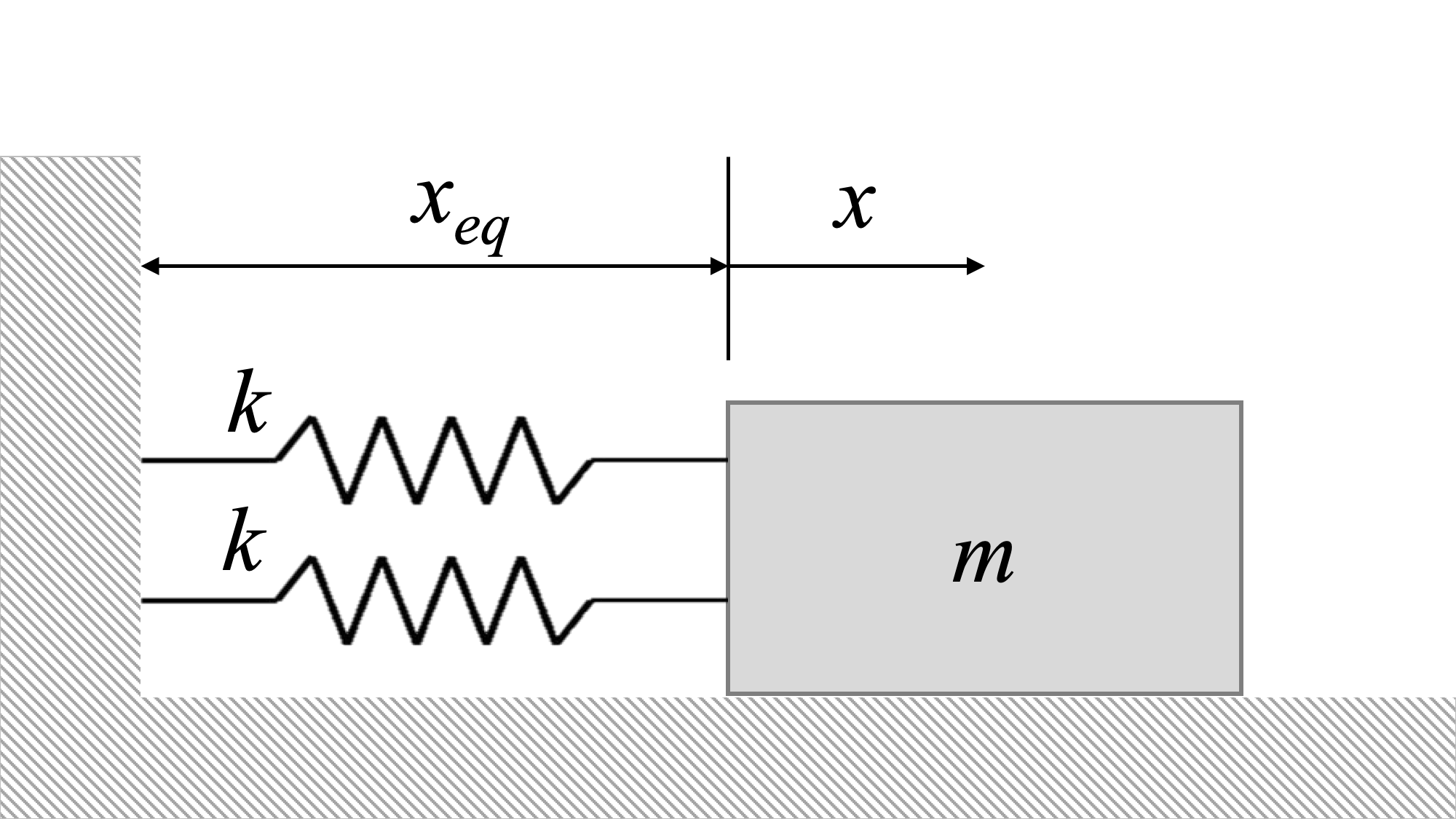### Question 2:

Determine the equation of motion of the system from Newton's Second Law. Assume mass m = 5 kg and spring constant k = 500 N/m. Find the initial displacement, x0, such that the mass oscillates over a total range of 4 m. Assume the initial perturbation velocity, v0, is 10 m/s.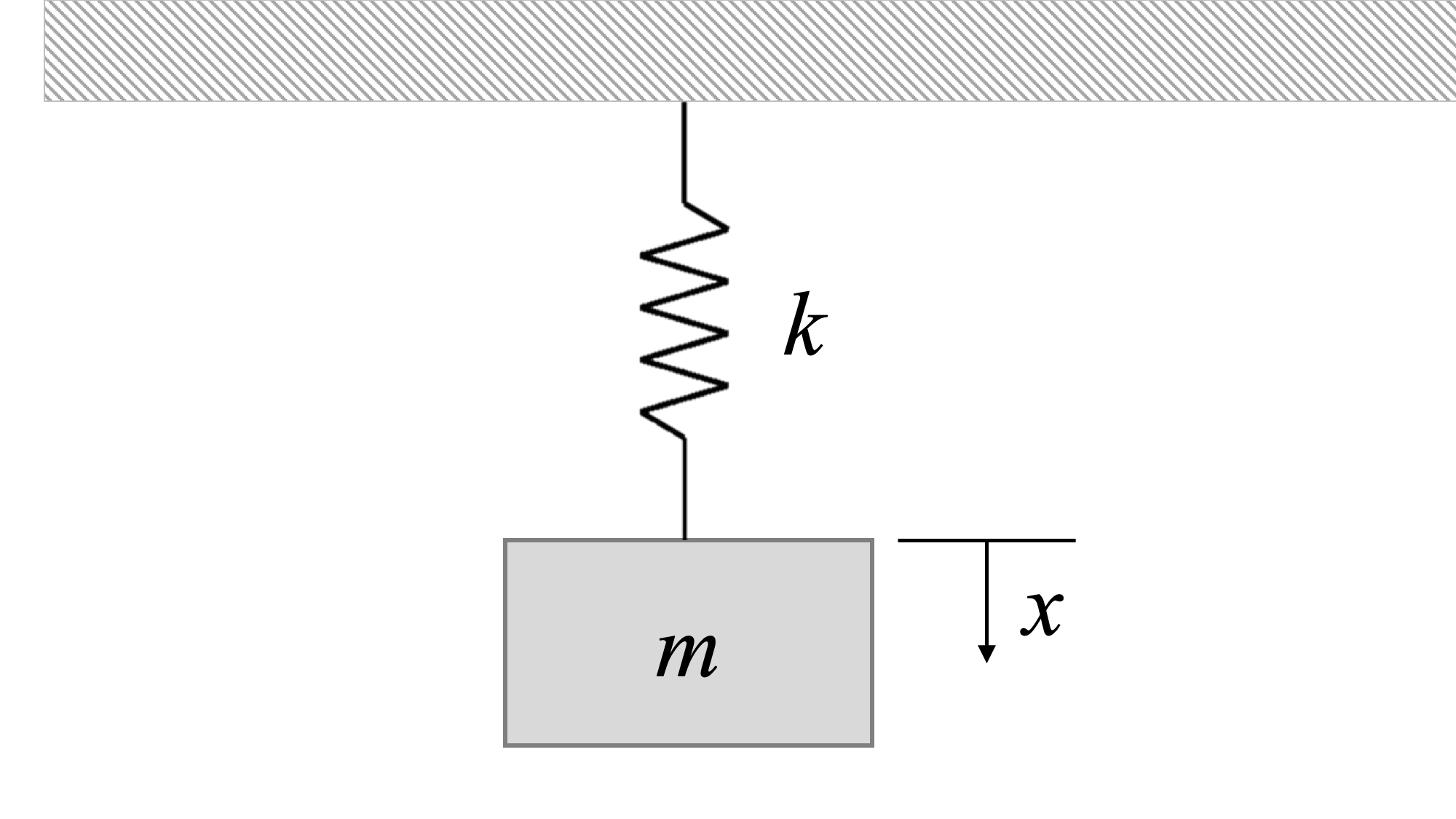### Question 3:

A target, with m=5kg, in an archery range is attached to a wall with a spring, k=50 N/m. An archer shoots a m=1kg arrow which sticks into the target. As a result of an impact with the arrow, the target has a horizontal initial velocity v_O=5m/s. Determine the maximum amplitude and the displacement at t=10s.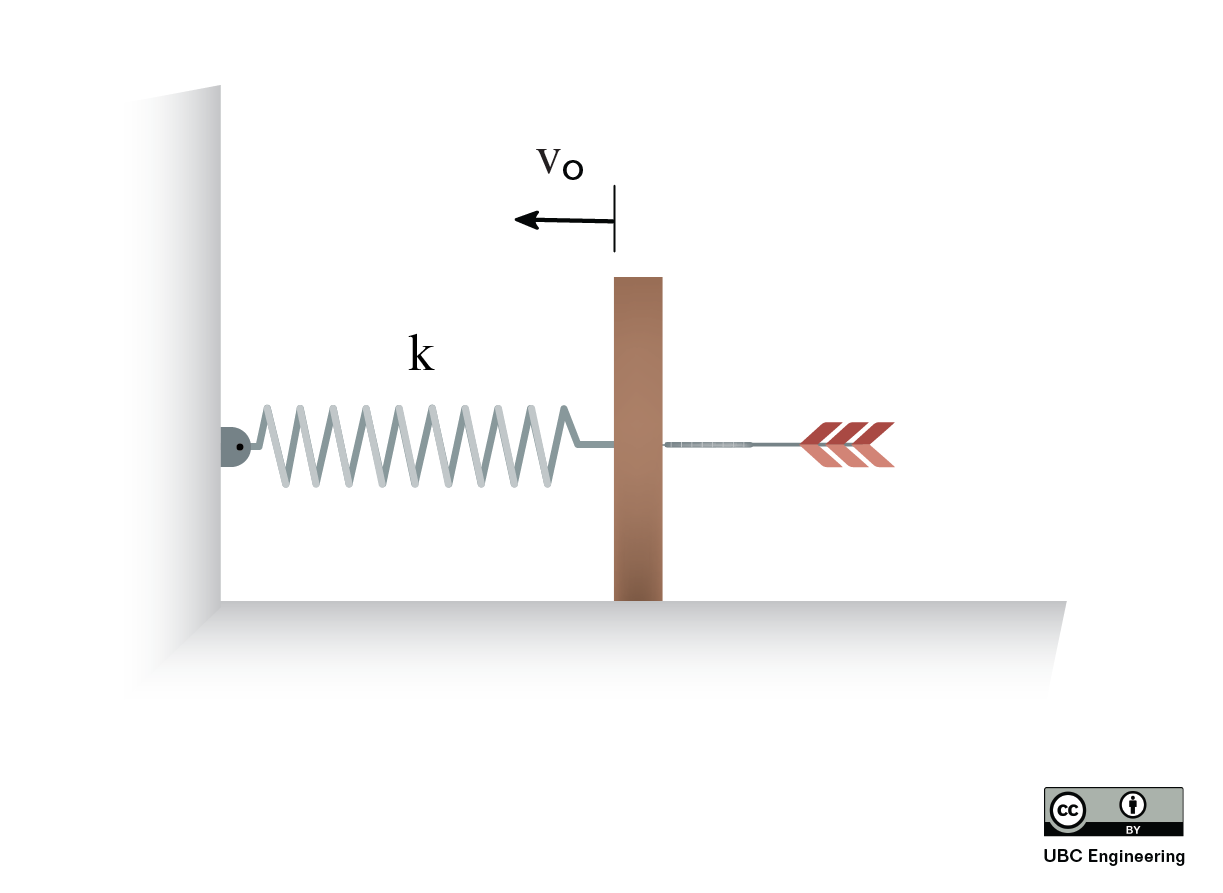### Question 4:

An equilateral triangle is made up of three individual bars. Each bar is 3m in length and 2kg in mass. Given that the frame is hung on the ceiling by one of its corners, determine the natural frequency.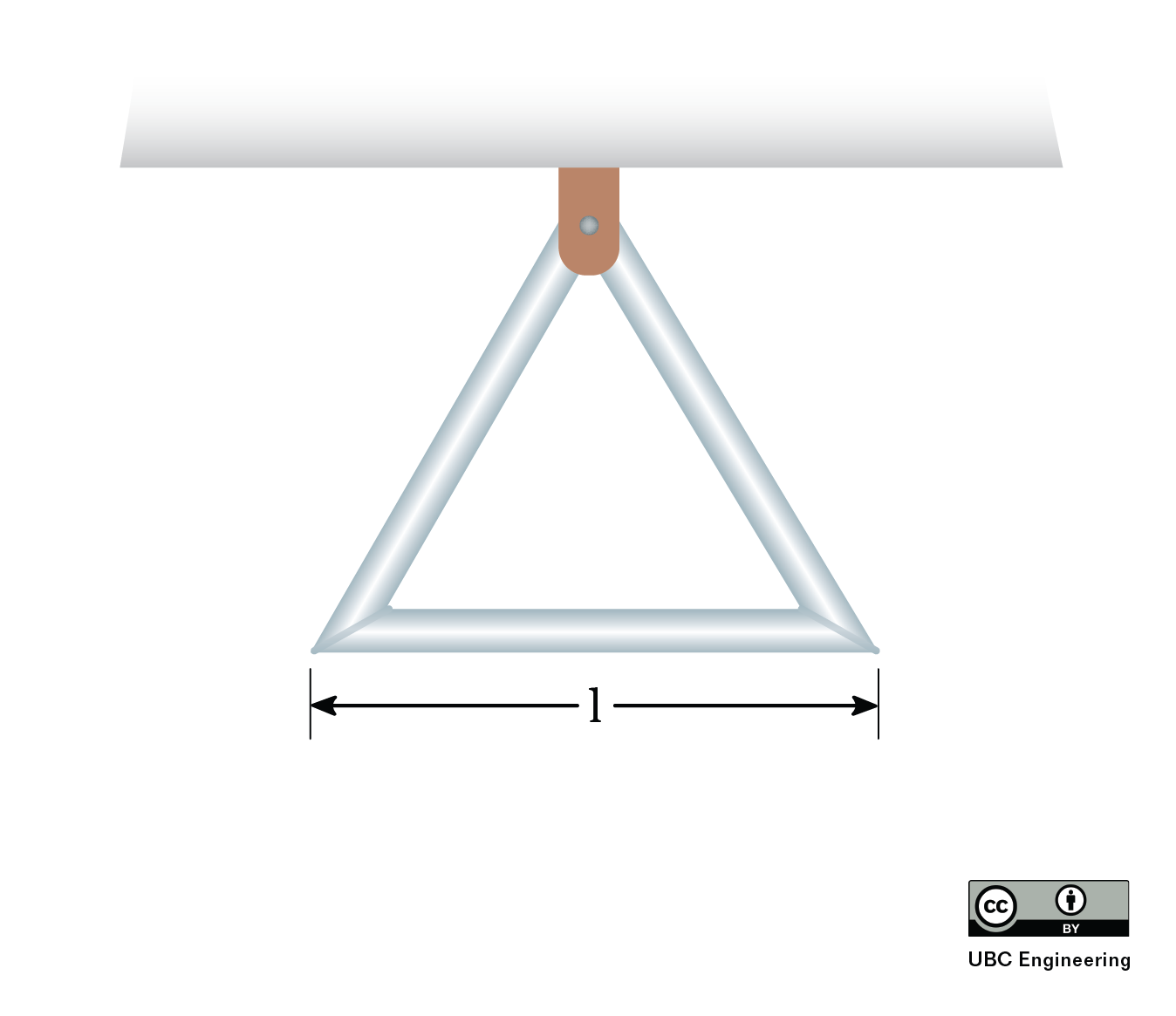### Question 5:

Find the natural angular frequency, ωn, for the system shown. The pulleys are frictionless and have negligible mass. Values are: m = 8 kg, k1= 300 N/m, k2 = 400 N/m.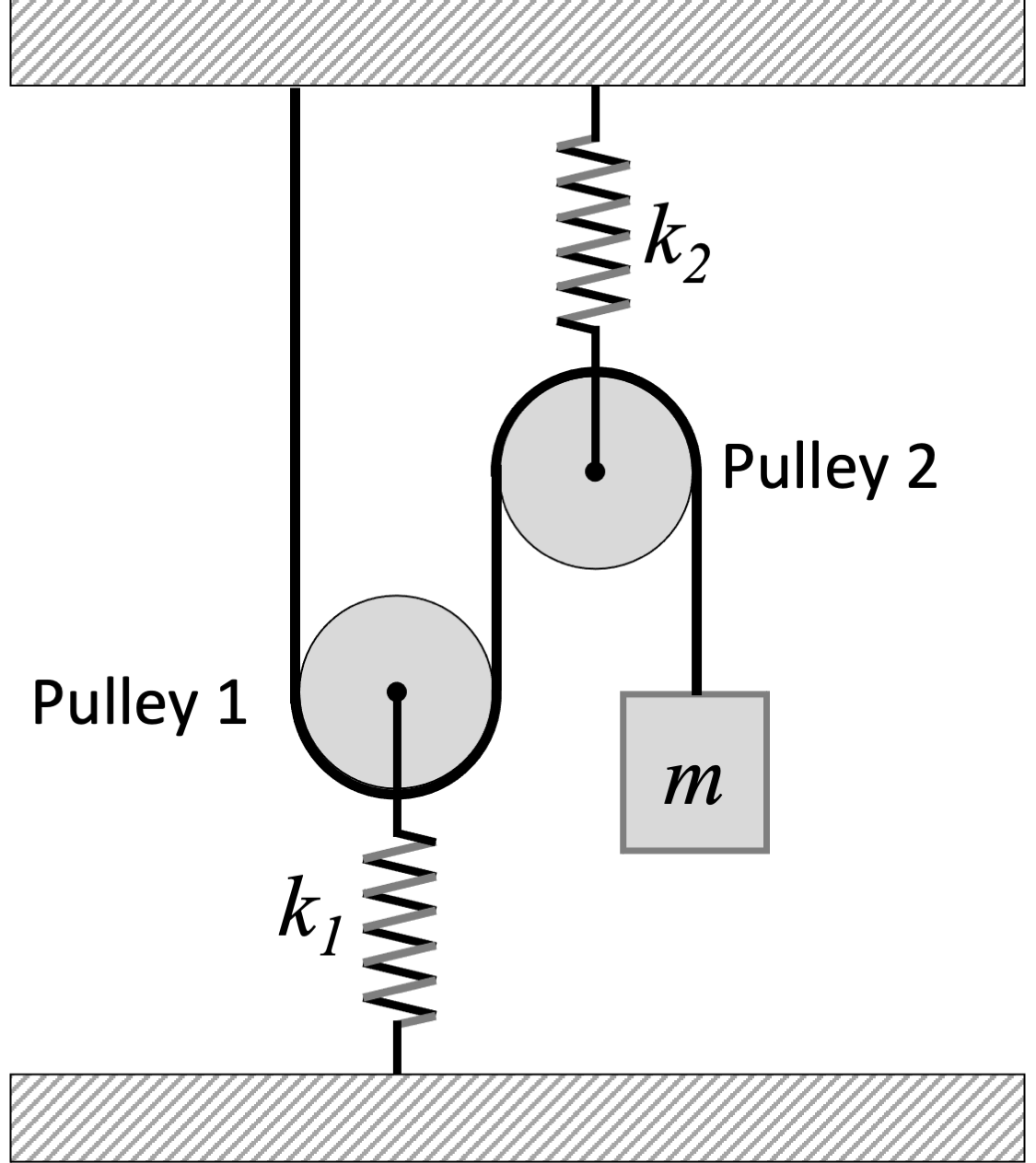### Question 6:

A spring with spring constant k = 90 N/m is connected to a disk with a mass 15 kg and radius r = 1 m. A moment of M= −40kˆ N · m is required to keep the disk in static equilibrium. At time t = t0 the moment is suddenly removed, and the disk begins to experience oscillatory motion due to the spring. With what period, τ, does the system oscillate? (You may assume sin θ = θ)